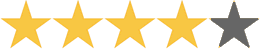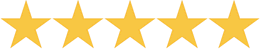A Beka Book Arithmetic 4 The colorful daily worksheets provide practice over familiar concepts and reteach the new material that the teacher has just taught. The four basic processes are taught and reviewed, as well as multiplying and dividing by two-digit numbers, estimation, square measures, writing decimals as fractions, and simple geometry. A major emphasis is on working with fractions, both proper and improper, adding subtracting, and multiplying fractions, and finding the least common denominator.
 Borenson & Associates Hands-on Equations Learning System Mary Pride says: ''Here's something really new-a hands-on approach to algebraic linear equations! You know what those are: fun stuff like 2x -2(-x+4)=x +(-2). With Hands-On Equations, learning pre-algebra and solving simple linear equations can be as fun and easy as playing a game.'' This patented teaching method empowers the teacher to successfully present essential algebraic concepts to students in grades 3 to adult. It does not require any algebraic prerequisites. Its system provides students with an intuitive understanding of the most fundamental algebraic concepts and makes algebraic linear equations accessible to 3rd grade gifted students, 4th grade average students, and 5th grade LD students. Hands-On Equations consists of: manuals for Levels I, II, & III; a student kit of game pieces with flat laminated balance; a set of worksheets; and an answer key.Price thisBob Jones University Press Math 4 Develops understanding of multiplication, division, decimals, and fraction concepts by using manipulatives. Includes an optional pre-algebra chapter, which introduces the addition and subtraction of integers. Continues to develop skills in estimating and problem solving. Teacher's Edition contains 165 lessons divided into 20 chapters. Includes teaching examples, skill practice activities, and a spiraling review to ensure retention of concepts. Provides additional information about teaching fact memorization as well as games and bulletin board ideas. Teacher's Edition: 920 pages; two spiral binders. Student Worktext features activities with Captain Bailey and Clipper, his sea gull, as they go ocean treasure hunting. Student Worktext: 344 pages; softbound; full color; perforated.
 Saxon Math 54 Excellent K-12 textbook and workbook math program. Students will generally work independently. Uses an effective incremental learning model.(4.2)
4 ReviewsPrice thisMath By Kids Written by Pennsylvania Homeschoolers. This unusual book of word problems was written by homeschool students of all ages. Arranged by categories - Math Problems About Food, Working and Earning, Mystery Numbers, Etc. Problems are listed from easiest to hardiest.Price thisMath Drillsters Bob Bernstein A reproducible activity book containing 220 pages of math drill activities primarily covering addition, subtraction, multiplication, division, but also touching on measurement, time, money, temperature, fractions and mathematical thinking.Price thisMath for Every Kid Janice VanCleave A supplemental math book for children ages 8-12 designed to make the learning of math skills a fun experience and to encourage a desire for further investigation into specific math topics.Price thisMathematicians are People, Too Luetta & Wilbert Reimer This 2 volume set contains 15 illustrated vignettes per book introducing students to great mathematicians from various cultures. Stories focus on moments of mathematical discovery and on personal anecdotes.(5)